Next: Photon statistics Up: Quantum statistics Previous: Formulation of the statistical

## Fermi-Dirac statistics

Let us, first of all, consider Fermi-Dirac statistics. According to Eq. (582), the average number of particles in quantum state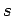can be written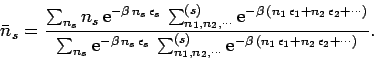(584)

Here, we have rearranged the order of summation, using the multiplicative properties of the exponential function. Note that the first sums in the numerator and denominator only involve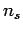, whereas the last sums omit the particular statefrom consideration (this is indicated by the superscripton the summation symbol). Of course, the sums in the above expression range over all values of the numbers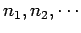such thatand 1 for each, subject to the overall constraint that(585)

Let us introduce the function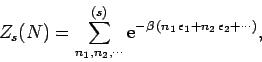(586)

which is defined as the partition function forparticles distributed over all quantum states, excluding state, according to Fermi-Dirac statistics. By explicitly performing the sum over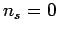and 1, the expression (584) reduces to(587)

which yields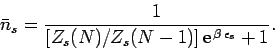(588)

In order to make further progress, we must somehow relateto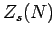. Suppose that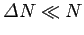. It follows thatcan be Taylor expanded to give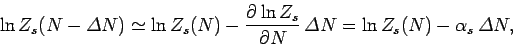(589)

where(590)

As always, we Taylor expand the slowly varying function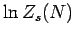, rather than the rapidly varying function, because the radius of convergence of the latter Taylor series is too small for the series to be of any practical use. Equation (589) can be rearranged to give(591)

Now, sinceis a sum over very many different quantum states, we would not expect the logarithm of this function to be sensitive to which particular stateis excluded from consideration. Let us, therefore, introduce the approximation that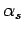is independent of, so that we can write(592)

for all. It follows that the derivative (590) can be expressed approximately in terms of the derivative of the full partition function(in which theparticles are distributed over all quantum states). In fact,(593)

Making use of Eq. (591), with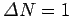, plus the approximation (592), the expression (588) reduces to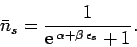(594)

This is called the Fermi-Dirac distribution. The parameteris determined by the constraint that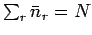: i.e.,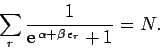(595)

Note thatifbecomes sufficiently large. On the other hand, since the denominator in Eq. (594) can never become less than unity, no matter how smallbecomes, it follows that. Thus,(596)

in accordance with the Pauli exclusion principle.

Equations (583) and (593) can be integrated to give(597)

where use has been made of Eq. (594).Next: Photon statistics Up: Quantum statistics Previous: Formulation of the statistical
Richard Fitzpatrick 2006-02-02